# Texas Go Math Grade 3 Lesson 11.1 Answer Key Write Related Facts

Refer to our Texas Go Math Grade 3 Answer Key Pdf to score good marks in the exams. Test yourself by practicing the problems from Texas Go Math Grade 3 Lesson 11.1 Answer Key Write Related Facts.

## Texas Go Math Grade 3 Lesson 11.1 Answer Key Write Related Facts

Essential Question
How can you write a set of related multiplication and division facts?
Related facts are set of addition and subtractions equations that involve the same numbers.
Related facts are also a set of multiplication and division equations that involve the same numbers.
Explanation:
For example;
2 + 3 = 5  and  5 – 3  = 2
8 ÷ 2 = 4  and 4 x 2 = 8
they are invers operations.

Unlock the Problem
Related facts are a set of related multiplication and division equations. What related facts can you write for 2, 4, and 8?
2 x 4 = 8
8÷ 4 = 2
Explanation:
As we know related facts are a set of related multiplication and division,
as they are inverse operations.

• What model can you use to show how multiplication and division are related?
we can write or use an array as a model to show how multiplication and division are related
the array can help us to write  related multiplication and division equations that contains related facts.

Activity
Materials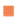square tiles

Step 1
Use 8 tiles to make an array with 2 equal rows. Draw the rest of the tiles.
How many tiles are in each row? ____
4 tiles in each row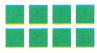Explanation:
we can write or use an array as a model to show how multiplication and division are related
the array can help us to write  related multiplication and division equations that contains related facts.
Draw  an array of total 8 tiles,
which are arranged in 2 equal rows.
8 ÷ 2 = 4
So, 4 tiles in each row.Explanation:
Write a division equation for the array using the total number of tiles as the dividend and the number of rows as the divisor.
___  ÷  ___ = ___
8 ÷ 2 = 4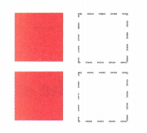Write a multiplication equation for the array.
___ × ___ = ____
8 ÷ 2 = 42 x 4 = 8Explanation:
Using 8 tiles an array is drawn.
Both multiplication and division facts are related to each other.

Step 2
Now, use 8 tiles to make an array with 4 equal rows.
Draw the rest of the tiles.
How many tiles are in each row? ___
Write a division equation for the array using the total number of tiles as the dividend and the number of rows as the divisor.
___ ÷ ___ = ___
So, 8 ÷ 2 = ____, 2 × 4 = ___, 8 ÷ 4 = ___ and 4 × 2 = ___ are related facts.Write a multiplication equation for the array.
___ × ___ =____
8 ÷ 2 = 4,2 × 4 =8,8 ÷ 4 =2 and 4 × 2 = 8 are related facts.Explanation:
we can write or use an array is a model to show how multiplication and division are related
the array can help us to write  related multiplication and division equations that contains related facts.

Try This! Draw an array with 4 rows of 4 tiles.
Your array shows the related facts for 4, 4, and 16.
4 × 4 = ___ 16 ÷ 4 = ___
Since both factors are the same, there are only two equations in this set of related facts.4 × 4 = 16 and  16 ÷ 4 = 4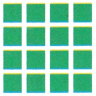Explanation:
Now, use 16 tiles to make an array with 4 equal rows.
Draw the rest of the tiles.
Each row has 4 tiles.
Related facts are also a set of multiplication and division equations that involve the same numbers.

Math Talk
Mathematical Processes
Look at the multiplication and division equations in a set of related facts. What do you notice about the products and dividends? Explain.

Question 1.
Complete the related facts for this array.2 × 8 = 16
______
16 ÷ 2 = 8
______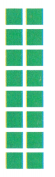Explanation:
An array is a model to show how multiplication and division are related,
the array can help us to write  related multiplication and division equations that contains related facts, both are inverse operations.

Write the related facts for the array.

Question 2.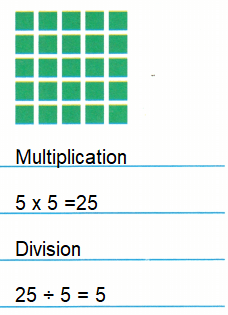Explanation:
As we know that multiplication and division are inverse operations.
With the help of array we can represent the related facts.

Question 3.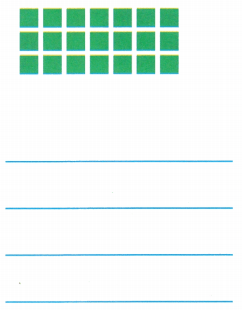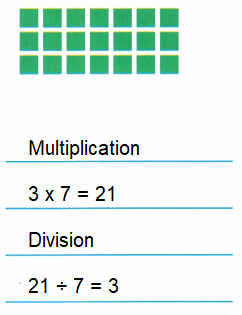Explanation:
As we know that multiplication and division are inverse operations.
With the help of array we can represent the related facts.

Question 4.Explanation:
Explanation:
As we know that multiplication and division are inverse operations.
With the help of array we can represent the related facts.

Complete the related facts.

Question 5.
4 × 7 = ____
7 × __ = 28
28 ÷ __ = 4
28 ÷ 4 = __
4 × 7 = 28
7 × 4 = 28
28 ÷ 7 = 4
28 ÷ 4 = 7
Explanation:
Division as the reverse operation of multiplication,
i.e. multiplication is about summing up equal groups of objects and
division is about dividing numbers of objects into equal sized groups.

Question 6.
5 × __ = 30
6 × __ = 30
30 ÷ 6 = ___
30 ÷ 5 = ___
5 × 6 = 30
6 × 5 = 30
30 ÷ 6 = 5
30 ÷ 5 = 6
Explanation:
Division as the reverse operation of multiplication,
i.e. multiplication is about summing up equal groups of objects and
division is about dividing numbers of objects into equal sized groups.

Question 7.
__ × 9 = 27
__ × 3 = 27
__ ÷ 9 = 3
27 ÷ ___ = 9
3 × 9 = 27
9 × 3 = 27
27÷ 9 = 3
27 ÷ 3 = 9
Explanation:
Division as the reverse operation of multiplication,
i.e. multiplication is about summing up equal groups of objects and
division is about dividing numbers of objects into equal sized groups.

Problem Solving

Use the table for 8-9.

Question 8.
HO.T. Multi-Step Mr. Lee divides 1 package of clay and 1 package of glitter dough equally among 4 students. How many more glitter dough sections than clay sections does each student get?6 more glitter dough sections than clay sections each student get.
Explanation:
Mr. Lee divides 1 package of clay = 12 sections
1 package of glitter dough = 36 sections
equally distributed among 4 students.
12 ÷ 4 = 3
36 ÷ 4 = 9
Number of more glitter dough sections than clay sections each student get
9 – 3 = 6

Question 9.
Write Math What’s the Error? Ty has a package of glitter dough. He says he can give 9 friends 5 equal sections. Explain his error.
Ty need 45 sections of glitter dough, but he has only 36 sections.
Explanation:
1 package of glitter dough = 36 sections
Ty want to distribute among 9 friends in 5 equal sections.
But 9 x 5 = 45
So, he cannot distribute equally.
Question 10.
H.O.T. Pose a Problem Write a word problem that can be solved by using 35 ÷ 5. Solve your problem.
A class has 5 students. Teacher want to distribute 35 markers among them equally. How many markers each student get?
Explanation:
Total markers = 35
Total students = 5
Number of markers each student get
35 ÷ 5 = 7

Question 11.
Write a set of related facts that has only two equations.
In math, a fact family can be defined as a group of math facts or equations created using the same set of numbers.
The fact family shows the relationships between the three numbers involved.
Similarly, in a multiplication and division fact family, there are multiplication and division sentences created using three numbers.
Explanation:
For example;
A fact family has been created using the numbers 12, 4 and 3 where 4 and 3 are parts, while 12 is the whole.Fill in the bubble for the correct answer choice.

Question 12.
There are 36 water bottles. Water bottles come in packages of 6. Which equation gives the related fact for the number of water bottles in each package?
(A) 36 ÷ 12 = 3
(B) 12 × 3 = 36
(C) 6 × 6 = 36
(D) 36 ÷ 9 = 4
Option(C)
Explanation:
There are 36 water bottles.
Water bottles come in packages of 6.
The number of water bottles in each package 6 x 6 = 36

Question 13.
Analyze Which equation is NOT included in the same set of related facts as 6 × 8 = 48?
(A) 48 ÷ 6 = 8
(B) 8 × 6 = 48
(C) 48 ÷ 8 = 6
(D) 48 ÷ 16 = 3
Option(D)
Explanation:
The fact family shows the relationships between the three numbers involved.
But in 48 ÷ 16 = 3 is not related in set of related facts.

Question 14.
Multi-Step Ryan and Madison are using an array to write equations. Ryan writes 6 × 2 = 12. Madison writes 12 ÷ 2 = 6. Which is a true statement about the equations?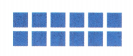(A) Both equations are correct.
(B) Only Ryan is correct.
(D) Both equations are incorrect.
Option (A)
Explanation:
Ryan writes 6 × 2 = 12.
Madison writes 12 ÷ 2 = 6.
The fact family shows the relationships between the three numbers involved,
as multiplication and division are inverse operations.
Texas Test Prep

Question 15.
Megan wrote an equation that is NOT included in the same set of related facts as 9 × 4 = 36. Which equation did Megan write?
(A) 4 × 9 = 36
(B) 36 ÷ 6 = 6
(C) 36 ÷ 4 = 9
(D) 36 ÷ 9 = 4
Option(B)
Explanation:
The fact family shows the relationships between the three numbers involved.
But in 36 ÷ 6 = 6 is not related in set of related facts.

### Texas Go Math Grade 3 Lesson 11.1 Homework and Practice Answer Key

Write the related facts for the array.

Question 1.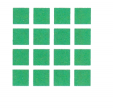4 x 4 = 16
16 ÷ 4 = 4
Explanation:
Division as the reverse operation of multiplication,
i.e. multiplication is about summing up equal groups of objects and
division is about dividing numbers of objects into equal sized groups.

Question 2.3 x 6 = 12
12 ÷ 3 = 6
Explanation:
Division as the reverse operation of multiplication,
i.e. multiplication is about summing up equal groups of objects and
division is about dividing numbers of objects into equal sized groups.

Complete the related facts.

Question 3.
___ × 4 = 32
___ × 8 = 32
____ ÷ 4 = 8
32 ÷ __ = 4
8 × 4 = 32
4 × 8 = 32
32 ÷ 4 = 8
32 ÷ 8 = 4
Explanation:
Multiplication and division are closely related,
given that division is the inverse operation of multiplication.
When we divide, we look to separate into equal groups,
while multiplication involves joining equal groups.

Question 4.
____ × 5 = 35
____ × 7 = 35
35 ÷ ___= 7
35 ÷ ___ = 5
7 × 5 = 35
5 × 7 = 35
35 ÷ 5 = 7
35 ÷ 7 = 5
Explanation:
Multiplication and division are closely related,
given that division is the inverse operation of multiplication.
When we divide, we look to separate into equal groups,
while multiplication involves joining equal groups.

Question 5.
7 × ___ = 63
9 × ___ = 63
___ ÷ 7 = 9
63 ÷ ___ = 7
7 × 9 = 63
9 × 7 = 63
63 ÷ 7 = 9
63 ÷ 9 = 7
Explanation:
Multiplication and division are closely related,
given that division is the inverse operation of multiplication.
When we divide, we look to separate into equal groups,
while multiplication involves joining equal groups.

Problem Solving

Question 6.
Ms. Cohn divides 21 markers equally among 7 students. Write an equation to show how many markers each student gets Then write a related fact.
3 markers
21 ÷ 7 = 3
Explanation:
Ms. Cohn divides 21 markers equally among 7 students.
Related fact equation is 21 ÷ 7 = 3
Each students get 3 markers.

Question 7.
Bethany makes an array of 5 rows of 5 tiles. What multiplication and related division equation can Bethany write about her array?
5 x 5 = 25
25 ÷ 5 = 5
Explanation:
Bethany makes an array of 5 rows of 5 tiles.
multiplication related equation 5 x 5 = 25
division related equation 25 ÷ 5 = 5

Lesson Check

Question 8.
Sheila has 24 coins in a coin book. The coins are in rows of 4. Which equation gives the related fact for the number of coins in each row?
(A) 24 ÷ 3 = 8
(B) 4 × 6 = 24
(C) 3 × 8 = 24
(D) 24 ÷ 8 = 3
Option (B)
Explanation:
Sheila has 24 coins in a coin book.
The coins are in rows of 4.
The number of coins in each row
24 ÷ 4 = 6

Question 9.
Jared has 4 boxes. He puts 9 toy cars in each box. Which equation gives the related fact for the number of toy cars Jared has in all?
(A) 36 = 9 × 4
(B) 36 = 6 × 6
(C) 36 ÷ 6 = 6
(D) 36 ÷ 3 = 12
Option(A)
Explanation:
Jared has 4 boxes.
He puts 9 toy cars in each box.
The number of toy cars Jared has in all 4 x 9 = 36

Question 10.
Which equation is NOT included in the same set of related facts as 7 × 6 = 42?
(A) 6 × 7 = 42
(B) 7 × 7 = 49
(C) 42 ÷ 6 = 7
(D) 42 ÷ 7 = 6
Option(B)
Explanation:
The fact family shows the relationships between the three numbers involved,
as multiplication and division are inverse operations.
But 7 × 7 = 49 equation is not related.

Question 11.
Which equation is NOT included in the same set of related facts as 6 × 9 = 54?
(A) 54 ÷ 6 = 9
(B) 9 × 6 = 54
(C) 54 ÷ 9 = 54
(D) 3 × 18 = 54
Option(D)
Explanation:
The fact family shows the relationships between the three numbers involved,
as multiplication and division are inverse operations.
But 3 × 18 = 54 equation is not related.

Question 12.
Multi-Step Enrico makes an array of 3 rows of 4 tiles. He writes 3 × 4 = 12 and 3 + 4 = 12. He says these are related facts. Which statement is true about Enrico’s equations?
(A) They are related facts.
(B) Only 3 × 4 is a related fact.
(C) Only 3 + 4 is a related fact.
(D) Both are not related facts.
Option(B)
Explanation:
Enrico makes an array of 3 rows of 4 tiles.
He writes 3 × 4 = 12 and 3 + 4 = 12.
But 3 + 4 = 12 is not correct as it is 7.

Question 13.
Multi-Step Maria makes an array of 4 rows of 5 tiles. She wants to make an array to show the fact 5 × 5. What can she do to change the array to show 5 × 5 as a related fact?
(A) Add one row of 5 tiles.
(B) Add 5 rows of 5 tiles.
(C) Take away one row of 5 tiles.